StatLect

Bernoulli distribution

Suppose you perform an experiment with two possible outcomes: either success or failure. Success happens with probability, while failure happens with probability. A random variable that takes valuein case of success andin case of failure is called a Bernoulli random variable (alternatively, it is said to have a Bernoulli distribution).Definition

Bernoulli random variables are characterized as follows.

Definition Letbe a discrete random variable. Let its support beLet. We say thathas a Bernoulli distribution with parameterif its probability mass function isA random variable having a Bernoulli distribution is also called a Bernoulli random variable.

Note that, by the above definition, any indicator function is a Bernoulli random variable.

The following is a proof thatis a legitimate probability mass function.

Proof

Non-negativity is obvious. We need to prove that the sum ofover its support equals. This is proved as follows:Expected value

The expected value of a Bernoulli random variableisProof

It can be derived as follows:Variance

The variance of a Bernoulli random variableisProof

It can be derived thanks to the usual variance formula ():Moment generating function

The moment generating function of a Bernoulli random variableis defined for any:Proof

Using the definition of moment generating function, we get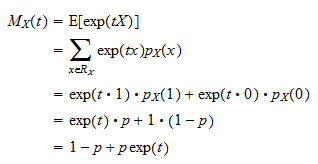Obviously, the above expected value exists for any.

Characteristic function

The characteristic function of a Bernoulli random variableisProof

Using the definition of characteristic function, we obtain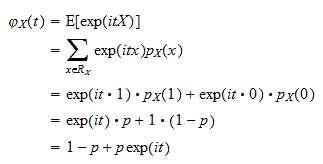Distribution function

The distribution function of a Bernoulli random variableis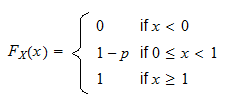Proof

Remember the definition of distribution function:and the fact thatcan take either valueor value. If, thenbecausecan not take values strictly smaller than. If, thenbecauseis the only value strictly smaller thanthatcan take. Finally, if, thenbecause all valuescan take are smaller than or equal to.

More details

Relation between the Bernoulli and the binomial distribution

A sum of independent Bernoulli random variables is a binomial random variable. This is discussed and proved in the lecture entitled Binomial distribution.

Solved exercises

Below you can find some exercises with explained solutions.

Exercise 1

Letandbe two independent Bernoulli random variables with parameter. Derive the probability mass function of their sumSolution

The probability mass function ofisThe probability mass function ofisThe support of(the set of valuescan take) isThe convolution formula for the probability mass function of a sum of two independent variables iswhereis the support of. When, the formula gives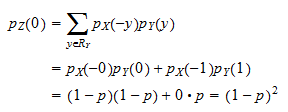When, the formula givesWhen, the formula gives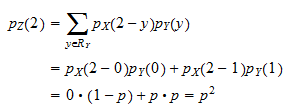Therefore, the probability mass function ofis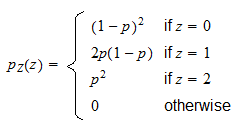Exercise 2

Letbe a Bernoulli random variable with parameter. Find its tenth moment.

Solution

The moment generating function ofisThe tenth moment ofis equal to the tenth derivative of its moment generating function, evaluated at: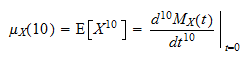Butso thatThe book

Most of the learning materials found on this website are now available in a traditional textbook format.

Glossary entries
Share